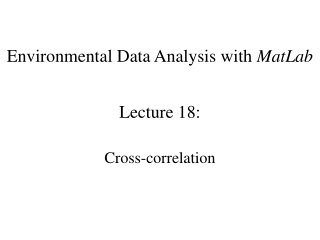DownloadDownload PresentationEnvironmental Data Analysis with MatLab

# Environmental Data Analysis with MatLab

Télécharger la présentation## Environmental Data Analysis with MatLab

- - - - - - - - - - - - - - - - - - - - - - - - - - - E N D - - - - - - - - - - - - - - - - - - - - - - - - - - -
##### Presentation Transcript

1. Environmental Data Analysis with MatLab Lecture 18: • Cross-correlation

2. SYLLABUS Lecture 01 Using MatLabLecture 02 Looking At DataLecture 03Probability and Measurement ErrorLecture 04 Multivariate DistributionsLecture 05Linear ModelsLecture 06 The Principle of Least SquaresLecture 07 Prior InformationLecture 08 Solving Generalized Least Squares ProblemsLecture 09 Fourier SeriesLecture 10 Complex Fourier SeriesLecture 11 Lessons Learned from the Fourier Transform Lecture 12 Power Spectral DensityLecture 13 Filter Theory Lecture 14 Applications of Filters Lecture 15 Factor Analysis Lecture 16 Orthogonal functions Lecture 17 Covariance and AutocorrelationLecture 18 Cross-correlationLecture 19 Smoothing, Correlation and SpectraLecture 20 Coherence; Tapering and Spectral Analysis Lecture 21 InterpolationLecture 22 Hypothesis testing Lecture 23 Hypothesis Testing continued; F-TestsLecture 24 Confidence Limits of Spectra, Bootstraps

3. purpose of the lecture generalize the idea of autocorrelation to multiple time series

4. Review of last lectureautocorrelationcorrelations between samples within a time series

5. high degree of short-term correlationwhat ever the river was doing yesterday, its probably doing today, toobecause water takes time to drain away

6. Neuse River Hydrograph A) time series, d(t) d(t), cfs time t, days

7. low degree of intermediate-term correlationwhat ever the river was doing last month, today it could be doing something completely differentbecause storms are so unpredictable

8. Neuse River Hydrograph A) time series, d(t) d(t), cfs time t, days

9. moderate degree of long-term correlationwhat ever the river was doing this time last year, its probably doing today, toobecause seasons repeat

10. Neuse River Hydrograph A) time series, d(t) d(t), cfs time t, days

11. 1 day 3 days 30 days

12. Autocorrelation Function 1 3 30

13. formula for covariance

14. formula for autocorrelation autocorrelation at lag (k-1)Δt

15. autocorrelation similar to convolution

16. autocorrelation similar to convolution note difference in sign

17. autocorrelation in MatLab

18. Important Relation #1autocorrelation is the convolution of a time series with its time-reversed self

19. Important Relationship #2Fourier Transform of an autocorrelationis proportional to thePower Spectral Density of time series

20. End of Review

21. Part 1correlations between time-series

22. scenariodischarge correlated with rainbut discharge is delayed behind rainbecause rain takes time to drain from the land

23. rain, mm/day time, days dischagre, m3/s time, days

24. rain, mm/day time, days rain ahead of discharge dischagre, m3/s time, days

25. rain, mm/day time, days shape not exactly the same, either dischagre, m3/s time, days

26. treat two time series u and v probabilistically p.d.f. p(ui, vi+k-1) with elements lagged by time (k-1)Δt and compute its covariance

27. this defines the cross-correlation

28. just a generalization of the auto-correlation different times in different time series different times in the same time series

29. like autocorrelation, similar to convolution

30. As with auto-correlationtwo important properties #1: relationship to convolution #2: relationship to Fourier Transform

31. As with auto-correlationtwo important properties #1: relationship to convolution #2: relationship to Fourier Transform cross-spectral density

32. cross-correlation in MatLab

33. Part 2aligning time-seriesa simple application of cross-correlation

34. central idea two time series are best alignedat the lag at which they are most correlated, which is the lag at which their cross-correlation is maximum

35. two similar time-series, with a time shift (this is simple “test” or “synthetic” dataset) u(t) v(t)

36. cross-correlate

37. find maximum maximum time lag

38. In MatLab

39. In MatLab compute cross-correlation

40. In MatLab compute cross-correlation find maximum

41. In MatLab compute cross-correlation find maximum compute time lag

42. align time series with measured lag u(t) v(t+tlag)

43. solar insolation and ground level ozone (this is a real dataset from West Point NY) A) B)

44. solar insolation and ground level ozone B) note time lag

45. maximum C) time lag 3 hours

46. A) B) original delagged